## Shell 语法错误，不知道怎么解决，程序很短，不到10行，求助

diffs.sh: line 7: syntax error near unexpected token `('
diffs.sh: line 7: `    diff <(sort f1)  <(sort f2)'

```#!/bin/bash
function diffs() {

echo "-----diff-----"
f1=\$1
f2=\$2
diff <(sort f1)  <(sort f2)

}
diffs f1 f2```

### 以下是问题补充：

@李东亮：用bash运行改正后的就行了。不能用sh (2013/08/13 17:53)

0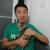```#!/bin/bash
f1=\$1
f2=\$2
function diffs() {
echo "-----diff-----"
diff <(sort \$f1)  <(sort \$f2)

}
diffs f1 f2```
0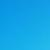#!/bin/bash
function diffs() {

echo "-----diff-----"
f1=\$1
f2=\$2
diff <(sort \$f1)  <(sort \$f2)

}
diffs \$1 \$2

0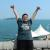#### 引用来自“holyzhou”的答案

#!/bin/bash
function diffs() {

echo "-----diff-----"
f1=\$1
f2=\$2
diff <(sort \$f1)  <(sort \$f2)

}
diffs \$1 \$2

diffs.sh: line 7: syntax error near unexpected token `('

diffs.sh: line 7: `    diff <(sort \$f1)  <(sort \$f2)'

0#### 引用来自“holyzhou”的答案

#!/bin/bash
function diffs() {

echo "-----diff-----"
f1=\$1
f2=\$2
diff <(sort \$f1)  <(sort \$f2)

}
diffs \$1 \$2

diffs.sh: line 7: syntax error near unexpected token `('

diffs.sh: line 7: `    diff <(sort \$f1)  <(sort \$f2)'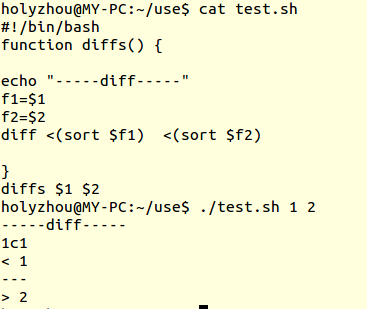0#### 引用来自“holyzhou”的答案

#!/bin/bash
function diffs() {

echo "-----diff-----"
f1=\$1
f2=\$2
diff <(sort \$f1)  <(sort \$f2)

}
diffs \$1 \$2

diffs.sh: line 7: syntax error near unexpected token `('

diffs.sh: line 7: `    diff <(sort \$f1)  <(sort \$f2)'0#### 引用来自“holyzhou”的答案

#!/bin/bash
function diffs() {

echo "-----diff-----"
f1=\$1
f2=\$2
diff <(sort \$f1)  <(sort \$f2)

}
diffs \$1 \$2

0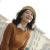```#!/bin/bash
diffs() {

echo '-----diff-----'
f1=\$1
echo \${f1}
f2=\$2
echo \${f2}
diff <(sort \${f1})  <(sort \${f2})
echo "diff <(sort \${f1})  <(sort \${f2})"

}
diffs f1 f2```

0
m

diff <(sort "\$f1")  <(sort "\$f2)，里面的"<()"是一个比较少见的特性：Process Substitution，这个功能不属于POSIX，在目前常用的Shell里，只有bash和zsh支持。

0#### 引用来自“holyzhou”的答案

#!/bin/bash
function diffs() {

echo "-----diff-----"
f1=\$1
f2=\$2
diff <(sort \$f1)  <(sort \$f2)

}
diffs \$1 \$2

diffs.sh: line 7: syntax error near unexpected token `('

diffs.sh: line 7: `    diff <(sort \$f1)  <(sort \$f2)'